International Tables for Crystallography (2006). Vol. D, ch. 1.6, pp. 150-177https://doi.org/10.1107/97809553602060000633

## Contents

• 1.6. Classical linear crystal optics  (pp. 150-177)
• 1.6.1. Introduction  (p. 150) | html | pdf |
• 1.6.2. Generalized optical, electro-optic and magneto-optic effects  (pp. 150-152) | html | pdf |
• 1.6.2.1. Spontaneous polarization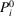(pp. 150-151) | html | pdf |
• 1.6.2.2. Dielectric polarization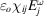(p. 151) | html | pdf |
• 1.6.2.3. Optical rotation (gyration)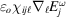(p. 151) | html | pdf |
• 1.6.2.4. Quadratic electric effect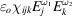(p. 151) | html | pdf |
• 1.6.2.5. Linear electro-optic effect(p. 151) | html | pdf |
• 1.6.2.6. Sum/difference frequency generation (two-wave mixing)(p. 151) | html | pdf |
• 1.6.2.7. Quadratic electro-optic effect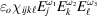(p. 151) | html | pdf |
• 1.6.2.8. Electric-field induced second harmonic generation(p. 151) | html | pdf |
• 1.6.2.9. Four-wave mixing(pp. 151-152) | html | pdf |
• 1.6.2.10. Faraday rotation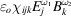(p. 152) | html | pdf |
• 1.6.2.11. Quadratic magneto-optic effect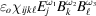(p. 152) | html | pdf |
• 1.6.2.12. Linear photoelastic effect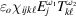(p. 152) | html | pdf |
• 1.6.2.13. Linear acousto-optic effect(p. 152) | html | pdf |
• 1.6.3. Linear optics  (pp. 152-154) | html | pdf |
• 1.6.3.1. The fundamental equation of crystal optics  (pp. 152-153) | html | pdf |
• 1.6.3.2. The optical indicatrix  (pp. 153-154) | html | pdf |
• 1.6.3.3. The dielectric impermeability tensor  (p. 154) | html | pdf |
• 1.6.4. Practical observation of crystals  (pp. 154-166) | html | pdf |
• 1.6.4.1. The polarizing microscope  (pp. 154-155) | html | pdf |
• 1.6.4.2. Specimen preparation  (p. 155) | html | pdf |
• 1.6.4.3. The indicatrix as an aid to practical microscopy  (p. 155) | html | pdf |
• 1.6.4.4. Vibration directions  (pp. 155-156) | html | pdf |
• 1.6.4.5. Measuring refractive indices  (pp. 156-157) | html | pdf |
• 1.6.4.6. Determination of linear birefringence  (pp. 157-158) | html | pdf |
• 1.6.4.7. Identification of polarization colours  (p. 158) | html | pdf |
• 1.6.4.8. Fringe counting  (pp. 158-159) | html | pdf |
• 1.6.4.9. Fast and slow vibration directions  (pp. 159-160) | html | pdf |
• 1.6.4.10. Other methods of measuring birefringence  (p. 160) | html | pdf |
• 1.6.4.11. Interference figures  (pp. 160-161) | html | pdf |
• 1.6.4.12. Uniaxial figures  (pp. 161-162) | html | pdf |
• 1.6.4.13. Biaxial figures  (pp. 162-165) | html | pdf |
• 1.6.4.14. Orientation studies  (pp. 165-166) | html | pdf |
• 1.6.4.15. Absorption colours  (p. 166) | html | pdf |
• 1.6.4.16. Dispersion  (p. 166) | html | pdf |
• 1.6.5. Optical rotation  (pp. 166-172) | html | pdf |
• 1.6.5.1. Introduction  (pp. 166-167) | html | pdf |
• 1.6.5.2. The dielectric tensor and spatial dispersion  (pp. 167-168) | html | pdf |
• 1.6.5.3. Symmetry of effective dielectric tensor  (p. 168) | html | pdf |
• 1.6.5.4. Gyration tensor  (p. 168) | html | pdf |
• 1.6.5.5. Optical rotation along the optic axis of a uniaxial crystal  (pp. 168-170) | html | pdf |
• 1.6.5.6. Optical rotation perpendicular to the optic axis of a uniaxial crystal  (pp. 170-172) | html | pdf |
• 1.6.6. Linear electro-optic effect  (pp. 172-173) | html | pdf |
• 1.6.6.1. Primary and secondary effects  (p. 172) | html | pdf |
• 1.6.6.2. Example of LiNbO 3   (pp. 172-173) | html | pdf |
• 1.6.7. The linear photoelastic effect  (pp. 173-176) | html | pdf |
• 1.6.7.1. Introduction  (pp. 173-174) | html | pdf |
• 1.6.7.2. Spontaneous strain in BaTiO 3   (pp. 174-175) | html | pdf |
• 1.6.7.3. The acousto-optic effect  (pp. 175-176) | html | pdf |
• 1.6.8. Glossary  (p. 176) | html | pdf |
• References | html | pdf |
• Figures
• Fig. 1.6.3.1. The optical indicatrix  (p. 153) | html | pdf |
• Fig. 1.6.3.2. Positive and negative uniaxial indicatrix  (p. 153) | html | pdf |
• Fig. 1.6.3.3. Biaxial indicatrix, showing the two optic axes and corresponding circular cross sections  (p. 154) | html | pdf |
• Fig. 1.6.4.1. A thin section of a rock containing the minerals aegirine (elongated crystals) and eudyalite (the matrix) viewed in plane-polarized light in two positions at right angles to each other  (p. 155) | html | pdf |
• Fig. 1.6.4.2. Successive sections across the optical path of the microscope  (p. 157) | html | pdf |
• Fig. 1.6.4.3. Plot of retardation, R , versus wavelength, showing how polarization colours are formed  (p. 157) | html | pdf |
• Fig. 1.6.4.4. A quartz wedge between crossed Nicols  (p. 158) | html | pdf |
• Fig. 1.6.4.5. ( a ) Photomicrograph between crossed polars of a thin section of rock containing large olivine crystals set in a fine-grained matrix  (p. 158) | html | pdf |
• Fig. 1.6.4.6. Polarization colours versus thickness  (p. 159) | html | pdf |
• Fig. 1.6.4.7. Three birefringence images of industrial diamond viewed along  taken with the rotating analyser system  (p. 161) | html | pdf |
• Fig. 1.6.4.8. Formation of the interference figure  (p. 161) | html | pdf |
• Fig. 1.6.4.9. ( a ) Centred uniaxial optic axis figure of a crystal with low birefringence  (p. 162) | html | pdf |
• Fig. 1.6.4.10. ( a ) Vibration directions of an oblique ray passing through calcite, viewed relative to the indicatrix  (p. 162) | html | pdf |
• Fig. 1.6.4.11. ( a ) Centred optic axis figure of quartz (uniaxial)  (p. 163) | html | pdf |
• Fig. 1.6.4.12. ( a ) The Biot–Fresnel construction illustrated on the stereographic projection  (p. 163) | html | pdf |
• Fig. 1.6.4.13. ( a ) Illustration of the formation of crossed isogyres when the optic axial plane of a biaxial figure lies N–S  (p. 163) | html | pdf |
• Fig. 1.6.4.14. ( a ) Drawing of biaxial optic axis figures set in the 45° position with the optic axial plane set NW–SE  (p. 164) | html | pdf |
• Fig. 1.6.4.15. ( a ) Acute bisectrix figure of a biaxial crystal with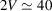°  (p. 164) | html | pdf |
• Fig. 1.6.5.1. Fresnel's explanation of optical rotation  (p. 167) | html | pdf |
• Fig. 1.6.5.2. Real and imaginary birefringence as a function of wavelength  (p. 167) | html | pdf |
• Fig. 1.6.5.3. The principle of superposition  (p. 170) | html | pdf |
• Fig. 1.6.6.1. ( a ) Symmetry elements of point group 3 m   (p. 173) | html | pdf |
• Fig. 1.6.7.1. ( a ) Symmetry elements of point group(p. 174) | html | pdf |
• Tables